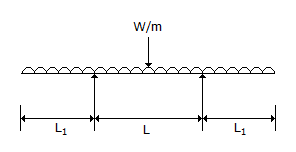# Civil Engineering - Strength of Materials - Discussion

### Discussion :: Strength of Materials - Section 3 (Q.No.14)

14.

For the beam shown in below figure, the maximum positive bending moment is nearly equal to negative bending moment when L1 is equal to[A]. 1.0 L [B]. 0.7 L [C]. 0.5 L [D]. 0.35 L.

Explanation:

No answer description available for this question.

 Powerexplorer said: (Jul 10, 2016) I think it's L1 = 0.21L.

 Siva said: (Sep 16, 2016) For me the answer is 0.207 L.

 Pavan said: (Sep 18, 2016) Ans D is correct. L1 = 0.207 (L + 2L1) (observe the length of beam those lengths are different from standard case). L1 = 0.35 L.

 Aspirant said: (Sep 18, 2016) Guys, do anyone knows which beam is this? Continues or overhanging.

 Shamsuddin said: (Sep 21, 2016) @Aspirant. It is Overhanging.

 Himzz The Great said: (Nov 25, 2016) No, answer is wrong I solved it and found out the answer is L1 = 1.207L and - .207L.

 Yuvarjsingh said: (Jul 4, 2017) Dear @@Aspirant. Continuous will always start with both ending supports, so it is overhanging.

 Gowthami.V said: (Jul 6, 2017) @PAVAN. How is it L1=0.207?

 Prasanta said: (Jul 19, 2017) Please describe the answer of this problem.

 A. Afarid said: (Aug 13, 2017) The corret option D.

 Priya said: (Jan 1, 2018) Please describe it briefly.

 Garry said: (Jun 23, 2018) Option D is correct the answer will be point 353 times L.

 Kajal Tomar said: (Jul 12, 2018) Correct ans is D; see how. Max. Positive B.M = W/8 ( L^2 - 4a^2). Max. Negative B.M = Wa^2 /2. W/8( L^2 - 4a^2 ) = Wa^2 /2 ( acc. to quest); Sove nd get a = 0.35 L. Here (L1 = a = overhang).

 Akshay said: (Sep 15, 2018) Thanks @Kajal.

 Anitha said: (Sep 24, 2020) @Kajal. How come 4a^2?

 Shubham said: (Jul 26, 2021) BM at centre = WL1^2/8 + wl1L1^2/2. Bm at support = WL1^2/2. WL1^2/8 + wl1L1^2/2 = WL1^2/2. = 0.207.

 Arun Kumar said: (Aug 20, 2021) For equal moment L1 = 0.35 L. For equal deflection L1 = 0.207 L.

 Arun Kumar said: (Aug 20, 2021) For equal moment L1 = 0.35 L, For max bending moment is minimum L1 = 0.207 L, If point load at both ends & for equal deflection condition L1= 0.207L.